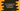# C++ program for Celsius to Fahrenheit and Fahrenheit to Celsius conversion## Introduction :

In this tutorial, we will learn how to convert a Celsius temperature value to Fahrenheit and vice versa. The program will take the inputs from the user and print out the result.

We are using the following two formulae :

``````fahrenheit = (1.8 * celsius) + 32

celsius = (fahrenheit - 32) / 32``````

The first one is used to convert a Celsius value to Fahrenheit and the second one is used to convert a Fahrenheit to Celsius.

The program will also ask the user to choose the conversion type at first i.e. Fahrenheit to Celsius or Celsius to Fahrenheit. Let’s take a look at the program :

### C++ program of temperature conversion :

``````#include <iostream>
using namespace std;

//1
int main()
{
//2
float fahrenheit, celsius;
int choice;

//3
cout << "Enter 1 : Fahrenheit to celsius \nEnter 2 : Celsius to Fahrenheit" << endl;
cin >> choice;

//4
if (choice == 1)
{
//5
cout << "Enter the Fahrenheit value :" << endl;
cin >> fahrenheit;

celsius = (fahrenheit - 32) / 1.8;
cout << "It is " << celsius << " degree celsius" << endl;
}
else if (choice == 2)
{
//6
cout << "Enter the Celsius value :" << endl;
cin >> celsius;

fahrenheit = (1.8 * celsius) + 32;
cout << "It is " << fahrenheit << " degree Fahrenheit" << endl;
}
else
{
//7
cout << "Please enter a valid input" << endl;
}
return 0;
}``````

### Explanation :

The commented numbers in the above program denote the step numbers below:

1. main() is the starting method of a C++ program. This method is called first.

2. We are creating two floating-point values to store the Fahrenheit and Celsius values. choice is an integer variable to store the option selected by the user.

3. Ask the user to enter 1 for Fahrenheit to Celsius and 2 for Celsius to Fahrenheit conversion. Read the user input value and store it in the choice variable.

4. Check the value of choice using an if-else if-else condition.

5. If the value of choice is 1, the conversion is for Fahrenheit to Celsius. Ask the user to enter the Fahrenheit value, store it in Fahrenheit variable and calculate the Celsius using the same formula we have seen above. Finally, print out the result to the user.

6. Similar to the above step, do the conversion from Celsius to Fahrenheit.

7. If the input is not valid, or if it is not either 1 or 2, print a message to the user.

### Sample Output :

``````Enter 1 : Fahrenheit to celsius
Enter 2 : Celsius to Fahrenheit
1
Enter the Fahrenheit value :
62
It is 16.6667 degree celsius

Enter 1 : Fahrenheit to celsius
Enter 2 : Celsius to Fahrenheit
2
Enter the Celsius value :
16.6667
It is 62.0001 degree Fahrenheit
``````

### Conclusion :

In this tutorial, we have learned how to convert a temperature in C++. Try to go through the program and drop one comment below if you have any queries.